4.1 Vocabulary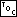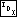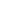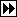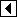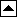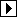When binary search trees, or BSTs, were introduced in the previous chapter, the reason that they were called binary search trees wasn't explained. The diagram below should help to clear up matters, and incidentally let us define some BST-related vocabulary:

This diagram illustrates the binary search tree example from the previous chapter. The circle or node (see node) at the top, labeled 107, is the starting point of any search. As such, it is called the root (see root) of the tree. The node connected to it below to the left, labeled 103, is the root's left child (see left child), and node 111 to its lower right is its right child (see right child). A node's left child corresponds to smaller from the array-based BST of the previous chapter, and a right child corresponds to larger.

Some nodes, such as 106 here, don't have any children. Such a node is called a leaf (see leaf) or terminal node (see terminal node). Although not shown here, it's also possible for a node to have only one child, either on the left or the right side. A node with at least one child is called a nonterminal node (see nonterminal node).

Each node in a binary search tree is, conceptually, the root of its own tree. Such a tree is called a subtree (see subtree) of the tree that contains it. The left child of a node and recursively all of that child's children is a subtree of the node, called the left subtree (see left subtree) of the node. The term right subtree (see right subtree) is defined similarly for the right side of the node. For instance, above, nodes 104, 105, and 106 are the right subtree of node 103, with 105 as the subtree's root.

A BST without any nodes is called an empty tree (see empty tree). Both subtrees of all even-numbered nodes in the BST above are empty trees.

In a binary search tree, the left child of a node, if it exists, has a smaller value than the node, and the right child of a node has a larger value. The more general term binary tree (see binary tree), on the other hand, refers to a data structure with the same form as a binary search tree, but which does not necessarily share this property. There are also related, but different, structures simply called “trees”.

In this book, all our binary trees are binary search trees, and this book will not discuss plain trees at all. As a result, we will often be a bit loose in terminology and use the term “binary tree” or “tree” when “binary search tree” is the proper term.

Although this book discusses binary search trees exclusively, it is instructive to occasionally display, as a counterexample, a diagram of a binary tree whose nodes are out of order and therefore not a BST. Such diagrams are marked ** to reinforce their non-BST nature to the casual browser.

See also:  [Knuth 1997], section 2.3; [Knuth 1998b], section 6.2.2; [Cormen 1990], section 13.1; [Sedgewick 1998], section 5.4.